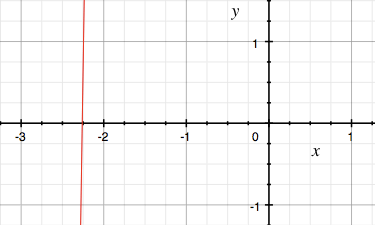SEARCH HOMEMath Central Quandaries & QueriesQuestion from Zuhdina, a student: Hi, I got a question, 2^x . 5^3 = x^4 I want to know the value of x without using calculator, I've already done some questions in logarithm and I always stuck in the same model equation-I think if someone shows me the way, I can do the other 'stuck equations'Hi Zuhdina,

Unfortunately there is no nice, neat algebraic solution to this problem. You mentioned logarithms and you can take the logarithm of each side to get

$x \log(2) + 3log(5) = 4 \log(x)$

which s no simpler than the equation you had to start. The best you can do is to approximate a solution.

My first step would e to define $f(x)$ by

$f(x) = 2^x \times 5^3 - x^4$

and use computer software or a graphing calculator to plot $y = f(x)$ and see where the graph crosses the x-axis. The graphing software on my computer gave meThe curve is in red so from the graph I can see that $f(x) = 0$ when x is approximately -2.25.

To obtain a better approximation I would use Wolfram Alpha. I typed in solve 2^x * 5^3 - x^4 = 0 and obtained $x = -2.26014.$

PennyMath Central is supported by the University of Regina and The Pacific Institute for the Mathematical Sciences.